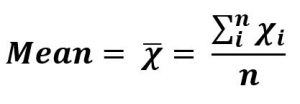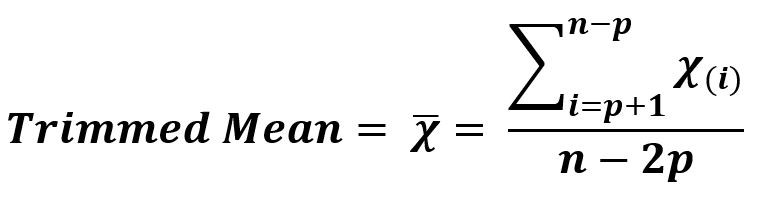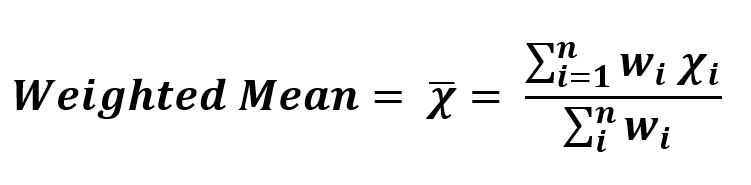# Understanding Types of Means | Set 1

• Last Updated : 30 Jul, 2021

It is one of the most important concepts of statistics, a crucial subject to learn Machine Learning.

• Arithmetic Mean : It is the mathematical expectation of a discrete set of numbers or average.
Denoted by , pronounced as “x-bar”. It is the sum of all the discrete values in the set divided by the total number of values in the set.
The formula to calculate the mean of n values – x1, x2, ….. xn• Example –
```Sequence = {1, 5, 6, 4, 4}

Sum             = 20
n, Total values = 5
Arithmetic Mean = 20/5 = 4```
• Code –

## Python3

 `# Arithmetic Mean`   `import` `statistics `   `# discrete set of numbers` `data1 ``=` `[``1``, ``5``, ``6``, ``4``, ``4``] `   `x ``=` `statistics.mean(data1) `   `# Mean ` `print``(``"Mean is :"``, x) `

• Output :
`Mean is : 4`
• Trimmed Mean : Arithmetic Mean is influenced by the outliers (extreme values) in the data. So, trimmed mean in used at the time of pre-processing when we are handling such kinds of data in machine learning.
It is arithmetic having a variation i.e. it is calculated by dropping a fixed number of sorted values from each end of the sequence of data given and then calculated the mean (average) of remaining values.• Example –

```Sequence = {0, 2, 1, 3}
p        = 0.25

Remaining Sequence  = {2, 1}
n, Total values = 2
Mean = 3/2 = 1.5```
• Code –

## Python3

 `# Trimmed Mean `   `from` `scipy ``import` `stats`   `# discrete set of numbers` `data ``=` `[``0``, ``2``, ``1``, ``3``] `   `x ``=` `stats.trim_mean(data, ``0.25``)`   `# Mean ` `print``(``"Trimmed Mean is :"``, x) `

• Output :
`Trimmed Mean is : 1.5`
• Weighted Mean : Arithmetic Mean or Trimmed mean is giving equal importance to all the parameters involved. But whenever we are working in machine learning predictions, there is a possibility that some parameter values hold more importance than the others, so we assign high weights to the values of such parameters. Also, there can be a chance that our data set has a highly variable value of a parameter, so we assign lesser weights to the values of such parameters.• Example –
```Sequence = [0, 2, 1, 3]
Weight   = [1, 0, 1, 1]

Sum (Weight * sequence)  = 0*1 + 2*0 + 1*1 + 3*1
Sum (Weight) = 3
Weighted Mean = 4 / 3 = 1.3333333333333333```
• Code 1 –

## Python3

 `# Weighted Mean `   `import` `numpy as np`   `# discrete set of numbers` `data ``=` `[``0``, ``2``, ``1``, ``3``] `   `x ``=` `np.average(data, weights ``=``[``1``, ``0``, ``1``, ``1``])`   `# Mean ` `print``(``"Weighted Mean is :"``, x) `

• Output 1 :
`Weighted Mean is : 1.3333333333333333`
• Code 2 –

## Python3

 `# Weighted Mean `   `data ``=` `[``0``, ``2``, ``1``, ``3``]` `weights ``=` `[``1``, ``0``, ``1``, ``1``]`   `x ``=` `sum``(data[i] ``*` `weights[i] ` `    ``for` `i ``in` `range``(``len``(data))) ``/` `sum``(weights)`     `print` `(``"Weighted Mean is :"``, x)`

• Output 2 :
`Weighted Mean is : 1.3333333333333333`

My Personal Notes arrow_drop_up
Recommended Articles
Page :